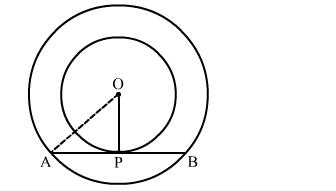# In two concentric circles, a chord of length 8 cm of the larger circle touches the smaller circle.`
Question:

In two concentric circles, a chord of length 8 cm of the larger circle touches the smaller circle. If the radius of the larger circle is 5 cm then Find the radius of the smaller circle.

Solution:We know that the radius and tangent are perperpendular at their point of contact
Since, the perpendicular drawn from the centre bisect the chord.

$\therefore \mathrm{AP}=\mathrm{PB}=\frac{\mathrm{AB}}{2}=4 \mathrm{~cm}$

In right  triangle AOP
AO2 = OP2 + PA2
⇒ 52 = OP2 + 42
⇒ OP2 = 9
⇒ OP = 3 cm
Hence, the radius of the smaller circle is 3 cm.